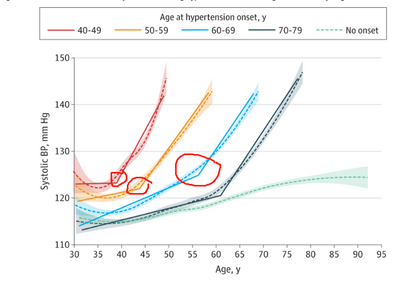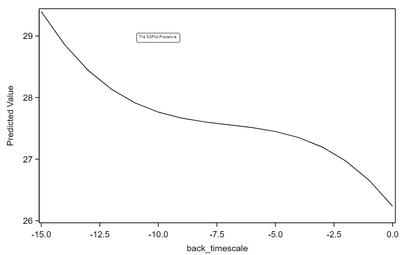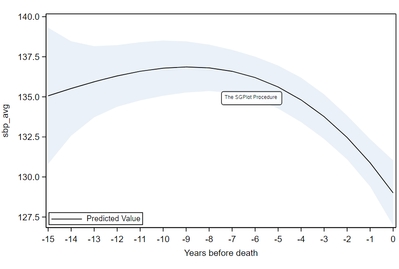## Detect inflection point or changing point

I know for a bell-shaped curve or a U-shaped curve, it is easy to find the changing point based on the model output from proc mixed by calculating the derivative.

However, if the derivatives for each point monotonously increase, e.g. for the blue line in the below picture, then there is no such extreme value.

How could we detect the changing point for those types of curves?1 ACCEPTED SOLUTION

Accepted Solutions

## Re: Detect inflection point or changing point

I'm not sure what data you have available. Are you saying that you have piecewise linear (PL) curves and you want to find the "elbow" points at which the slope changes? If so, read "Using finite differences to estimate the maximum of a time series." The article talks about using finite differences to estimate the maximum, which is defined as when the slope of the PL curve changes sign. However, you can use the same technique and the DIF function to find when the slope of a PL line changes slope:

``````data Have;
input x y;
datalines;
1 2
2 3
4 5
5 7
7 11
8 13
;

proc sgplot data=Have;
series x=x y=y / markers;
run;

data Want;
set Have;
slope = dif(y) / dif(x);  /* estimate the slope by using backward difference */
SlopeChanged = dif(slope);          /* indicate when the slope changes */
run;

proc print data=Want; run;
``````
8 REPLIES 8

## Re: Detect inflection point or changing point

I haven't done it myself in SAS, but it sounds like you might be looking for a spline model.

SAS has a few ways to do that, I believe, but the only procedure that comes to mind off the top of my head is PROC ADAPTIVEREG. https://documentation.sas.com/doc/en/pgmsascdc/9.4_3.4/statug/statug_odsgraph_sect109.htm

## Re: Detect inflection point or changing point

I'm not sure what data you have available. Are you saying that you have piecewise linear (PL) curves and you want to find the "elbow" points at which the slope changes? If so, read "Using finite differences to estimate the maximum of a time series." The article talks about using finite differences to estimate the maximum, which is defined as when the slope of the PL curve changes sign. However, you can use the same technique and the DIF function to find when the slope of a PL line changes slope:

``````data Have;
input x y;
datalines;
1 2
2 3
4 5
5 7
7 11
8 13
;

proc sgplot data=Have;
series x=x y=y / markers;
run;

data Want;
set Have;
slope = dif(y) / dif(x);  /* estimate the slope by using backward difference */
SlopeChanged = dif(slope);          /* indicate when the slope changes */
run;

proc print data=Want; run;
``````

## Re: Detect inflection point or changing point

The paper is very interesting.

## Re: Detect inflection point or changing point

Hi Rick,

The method you mention could deal with the graph with the changing sign of slopes, eg. from positive to negative.

However, some graphs in my example are without the changing direction of slopes. I am wondering how to detect the changing point in this situation.

Below is my dataset. X= back_timescale, y = predicted.

 Obs back_timescale Predicted Lower Upper slope SlopeChanged 1 -15 29.40 27.60 31.19 . . 2 -14 28.86 27.49 30.22 -0.54 . 3 -13 28.44 27.37 29.52 -0.42 0.12 4 -12 28.13 27.25 29.02 -0.31 0.11 5 -11 27.91 27.13 28.69 -0.22 0.09 6 -10 27.76 27.06 28.47 -0.15 0.07 7 -9 27.67 27.02 28.31 -0.10 0.05 8 -8 27.60 27.01 28.20 -0.06 0.04 9 -7 27.56 27.01 28.11 -0.05 0.02 10 -6 27.51 27.00 28.03 -0.05 0.00 11 -5 27.45 26.95 27.95 -0.06 -0.02 12 -4 27.35 26.85 27.85 -0.10 -0.04 13 -3 27.20 26.70 27.70 -0.15 -0.05 14 -2 26.97 26.47 27.47 -0.22 -0.07 15 -1 26.66 26.15 27.16 -0.31 -0.09 16 0 26.24 25.69 26.78 -0.42 -0.11

This is the graph between x and y.Since the back_timescale include limited point (-15 to 0 by 1 ), could I use different point as the spline point and then compare their fit statistics (AIC, BIC) to decide the best spline point?

## Re: Detect inflection point or changing point

I think your table shows the solution: Look for where SlopeChanged changes sign. That indicates that the graph is changing from concave up to concave down, or, equivalently, that the graph is at an inflection point. For your example, this happens at Obs=10.

## Re: Detect inflection point or changing point

Hi Rick,

There are some different situations.

For example, the below graph. Each time point is with a smaller slope compared with the previous one.Obs back_timescale Predicted Lower Upper slope SlopeChanged 1 -15 135.06 130.82 139.31 . . 2 -14 135.52 132.57 138.47 0.46 . 3 -13 135.94 133.72 138.16 0.42 -0.03 4 -12 136.30 134.38 138.23 0.37 -0.06 5 -11 136.59 134.78 138.41 0.29 -0.08 6 -10 136.79 135.06 138.51 0.19 -0.10 7 -9 136.87 135.27 138.46 0.08 -0.12 8 -8 136.81 135.36 138.26 -0.06 -0.14 9 -7 136.59 135.25 137.93 -0.21 -0.16 10 -6 136.20 134.90 137.51 -0.39 -0.18 11 -5 135.61 134.27 136.95 -0.59 -0.20 12 -4 134.81 133.42 136.19 -0.81 -0.22 13 -3 133.76 132.37 135.16 -1.04 -0.24 14 -2 132.46 131.09 133.83 -1.30 -0.26 15 -1 130.88 129.40 132.36 -1.58 -0.28 16 0 129.01 126.97 131.04 -1.88 -0.30

## Re: Detect inflection point or changing point

What are you trying to do?

If you want to find a maximum or minumum, then look at where the slope changes sign. In calculus, this is called the First Derivative Test for a local extremum.

If you have a monotonic curve, the curve can change concavity. You can use the Second Derivative Test to determine if a curve is concave up or concave down at a point. If there is a point at which the first derivative is zero and the second derivative changes sign, then that point is called an inflection point

A curve can change concavity without having an inflection point. And, of course, some monotonic curves do not change concavity, such as y=x^2 or y=exp(x).